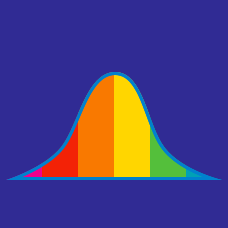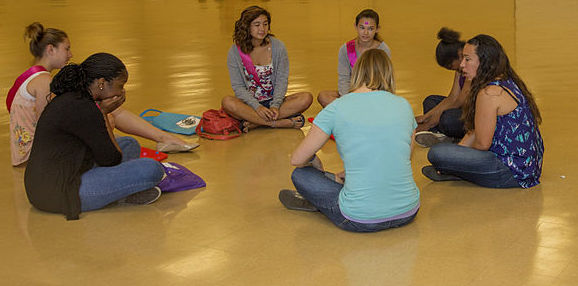Probability

# Understanding Data - Problem Solving

In Mrs Krol's math class, there are 20 students and the class average is 91. In Mr Tan's math class, there are 30 students and the class average is 71. What is the average of score of the combined classes?

Let the median of $33$ observations be $50.$ If each of the observations greater than the median is increased by $8,$ then what is the median of the new data?

$\{ -3.3, 6.7, x, 29.2, 42.4 \}$

How many values of $x$ are there that would make the range of the above set equal to 50?

A set of three distinct positive integers has mean 4 and median 5. What is the largest number in the set?

Details and assumptions

The mean of a set of numbers is the average of the set.

The median of a set of numbers is the middle value, which divides the list into two equal halves. If there is an even number of them, the median will be the average of the two middle values.A group of 7 girls each wrote down a number The average of the 7 numbers is 50.
The average of the numbers not written by Sally is 45.
What number did Sally write?

×